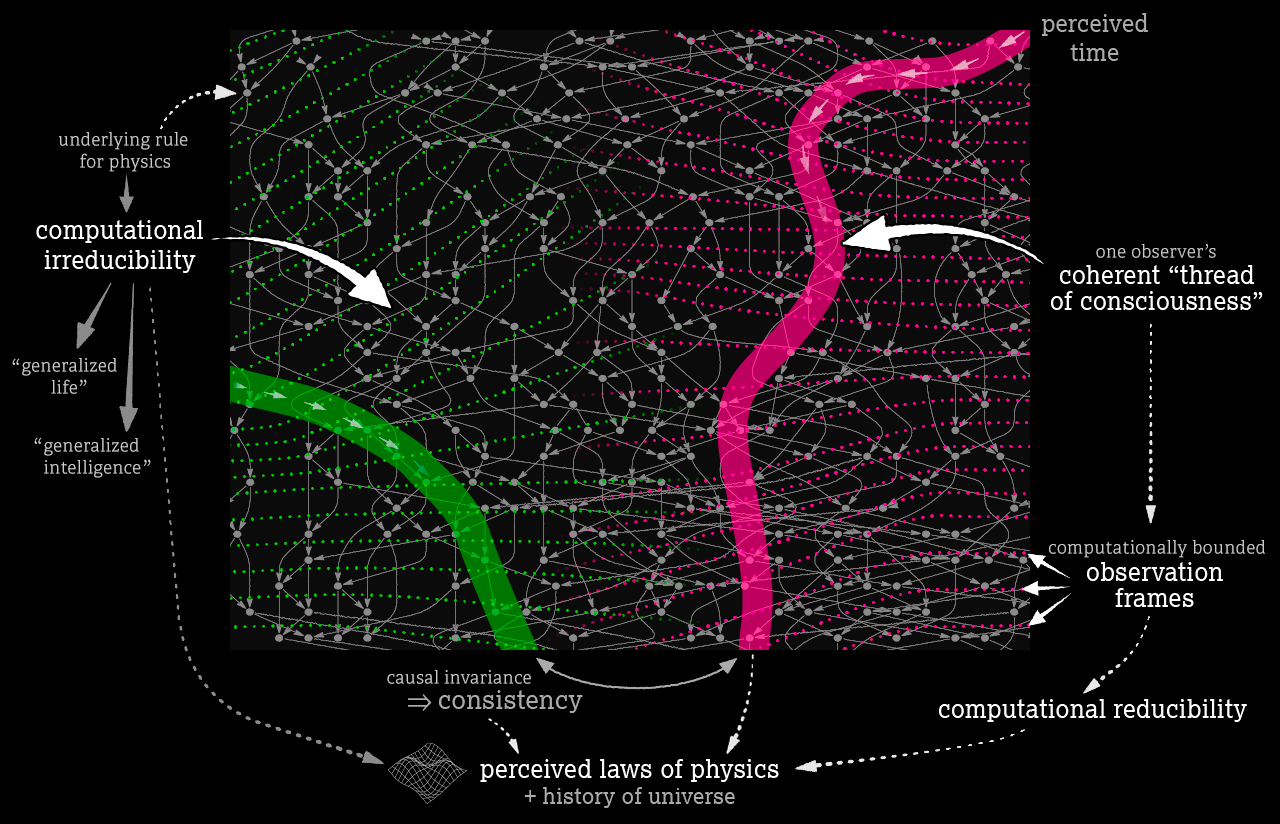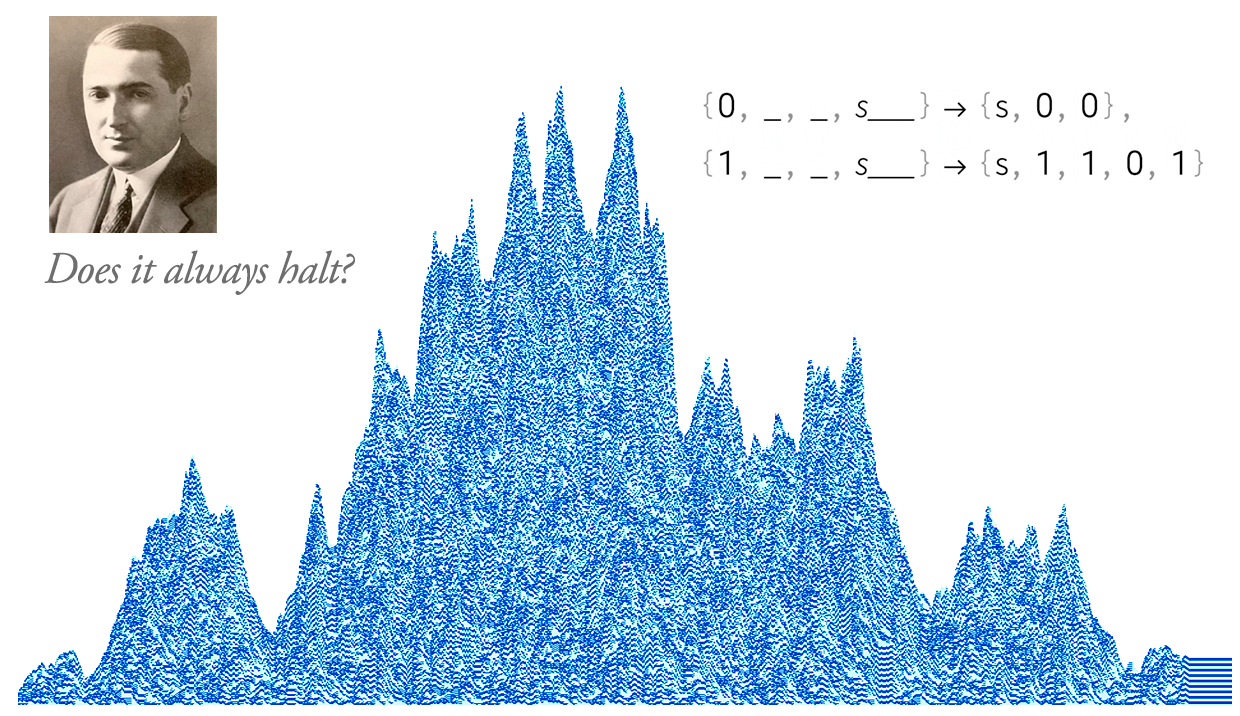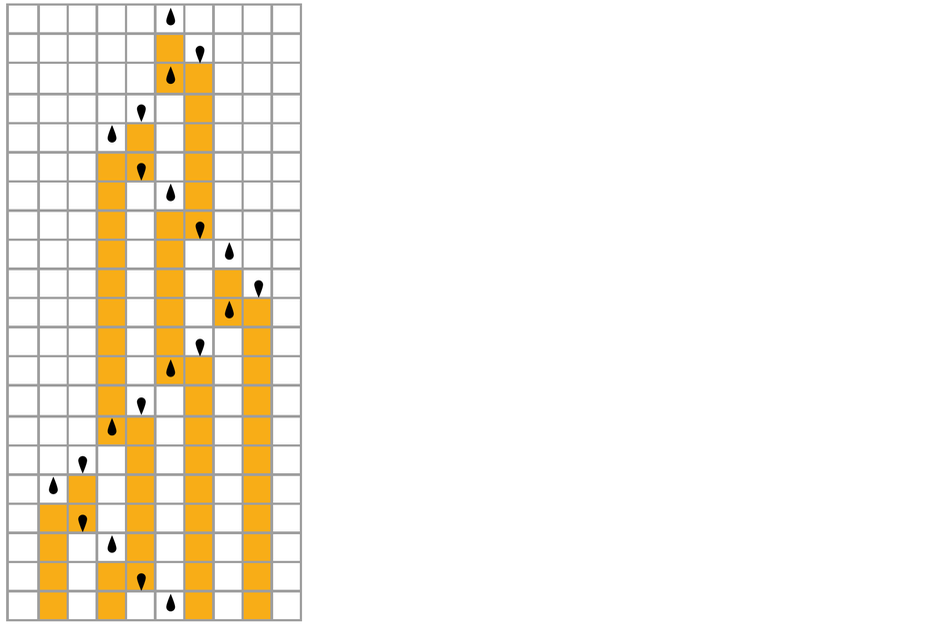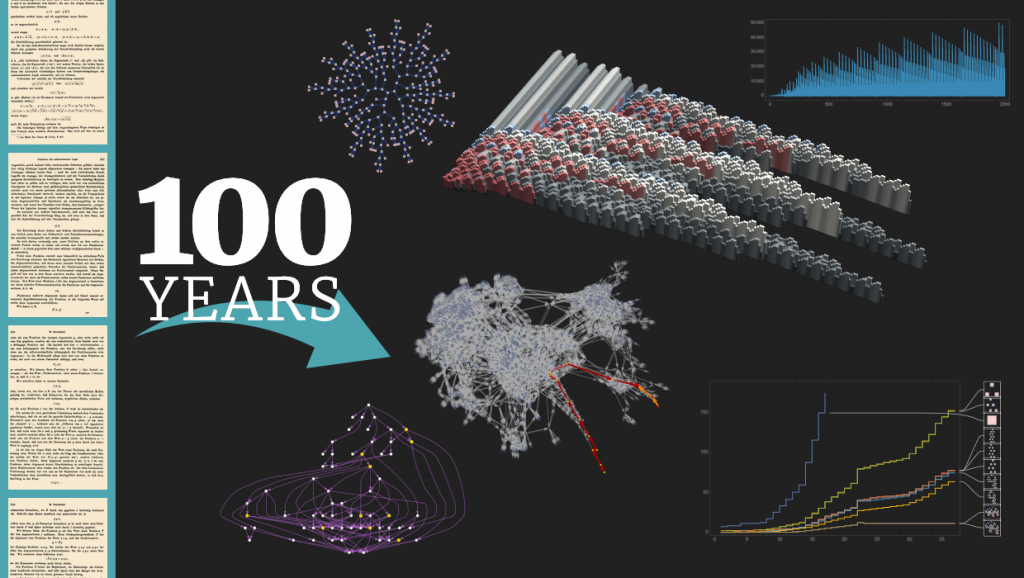# ZX-Calculus and Extended Wolfram Model Systems II: Fast Diagrammatic Reasoning with an Application to Quantum Circuit Simplification

This article presents a novel algorithmic methodology for performing automated diagrammatic deductions over combinatorial structures, using a combination of modified equational theorem-proving techniques and the extended Wolfram model hypergraph rewriting formalism developed by the authors in previous work. We focus especially upon the application of this new algorithm to the problem of automated circuit simplification in quantum information theory, using Wolfram model multiway operator systems combined with the ZX-calculus formalism for enacting fast diagrammatic reasoning over linear transformations between qubits. We show how to construct a generalization of the deductive inference rules for Knuth-Bendix completion in which equation matches are selected on the basis of causal edge density in the associated multiway system, before proceeding to demonstrate how to embed the higher-order logic of the ZX-calculus rules within this first-order equational framework. After showing explicitly how the (hyper)graph rewritings of both Wolfram model systems and the ZX-calculus can be effectively realized within this formalism, we proceed to exhibit comparisons of time complexity vs. proof complexity for this new algorithmic approach when simplifying randomly-generated Clifford circuits down to pseudo-normal form, as well as when reducing the number of T-gates in randomly-generated non-Clifford circuits, with circuit sizes ranging up to 3000 gates, illustrating that the method performs favorably in comparison with existing circuit simplification frameworks, and also exhibiting the approximately quadratic speedup obtained by employing the causal edge density optimization. Finally, we present a worked example of an automated proof of correctness for a simple quantum teleportation protocol, in order to demonstrate more clearly the internal operations of the theorem-proving procedure.

# What Is Consciousness? Some New Perspectives from Our Physics ProjectFor years I’ve batted it away. I’ll be talking about my discoveries in the computational universe, and computational irreducibility, and my Principle of Computational Equivalence, and people will ask “So what does this mean about consciousness?” And I’ll say “that’s a slippery topic”. And I’ll start talking about the sequence: life, intelligence, consciousness.

I’ll ask “What is the abstract definition of life?” We know about the case of life on Earth, with all its RNA and proteins and other implementation details. But how do we generalize? What is life generally? And I’ll argue that it’s really just computational sophistication, which the Principle of Computational Equivalence says happens all over the place. Then I’ll talk about intelligence. And I’ll argue it’s the same kind of thing. We know the case of human intelligence. But if we generalize, it’s just computational sophistication—and it’s ubiquitous. And so it’s perfectly reasonable to say that “the weather has a mind of its own”; it just happens to be a mind whose details and “purposes” aren’t aligned with our existing human experience.

# After 100 Years, Can We Finally Crack Post’s Problem of Tag? A Story of Computational Irreducibility, and More## “[Despite] Considerable Effort… [It Proved] Intractable”

In the early years of the twentieth century it looked as if—if only the right approach could be found—all of mathematics might somehow systematically be solved. In 1910 Whitehead and Russell had published their monumental Principia Mathematica showing (rather awkwardly) how all sorts of mathematics could be represented in terms of logic. But Emil Post wanted to go further. In what seems now like a rather modern idea (with certain similarities to the core structure of the Wolfram Language, and very much like the string multiway systems in our Physics Project), he wanted to represent the logic expressions of Principia Mathematica as strings of characters, and then have possible operations correspond to transformations on these strings.

In the summer of 1920 it was all going rather well, and Emil Post as a freshly minted math PhD from Columbia arrived in Princeton to take up a prestigious fellowship. But there was one final problem. Having converted everything to string transformations, Post needed to have a theory of what such transformations could do.

PRELIMINARY VERSION

# Hypergraph Discretization of the Cauchy Problem in General Relativity via Wolfram Model Evolution

Although the traditional form of the Einstein ﬁeld equations is intrinsically four-dimensional, the ﬁeld of numerical general relativity focuses on the reformulation of these equations as a 3 + 1-dimensional Cauchy problem, in which Cauchy initial data is speciﬁed on a three-dimensional spatial hypersurface, and then evolved forwards in time. The Wolfram model oﬀers an inherently discrete formulation of the Einstein ﬁeld equations as an a priori Cauchy problem, in which Cauchy initial data is speciﬁed on a single spatial hypergraph, and then evolved by means of hypergraph substitution rules, with the resulting causal network corresponding to the conformal structure of spacetime. This article introduces a new numerical general relativity code based upon the conformal and covariant Z4 (CCZ4) formulation with constraint-violation damping, with the option to reduce to the standard BSSN formalism if desired, with Cauchy data deﬁned over hypergraphs; the code incorporates an unstructured generalization of the adaptive mesh reﬁnement technique proposed by Berger and Colella, in which the topology of the hypergraph is reﬁned or coarsened based upon local conformal curvature terms. We validate this code numerically against a variety of standard spacetimes, including Schwarzschild black holes, Kerr black holes, maximally extended Schwarzschild black holes, and binary black hole mergers (both rotating and non-rotating), and explicitly illustrate the relationship between the discrete hypergraph topology and the continuous Riemannian geometry that is being approximated. Finally, we compare the results produced by this code to the results obtained by means of pure Wolfram model evolution (without the underlying PDE system), using a hypergraph substitution rule that provably satisﬁes the Einstein ﬁeld equations in the continuum limit, and show that the two sets of discrete spacetimes converge to the same limiting geometries.

PRELIMINARY VERSION

# Video work logs

Over the years I’ve studied the simplest ordinary Turing machines quite a bit, but I’ve barely looked at multiway Turing machines (also known as nondeterministic Turing machines or NDTMs). Recently, though, I realized that multiway Turing machines can be thought of as “maximally minimal” models both of concurrent computing and of the way we think about quantum mechanics in our Physics Project. So now this piece is my attempt to “do the obvious explorations” of multiway Turing machines. And as I’ve found so often in the computational universe, even cases with some of the very simplest possible rules yield some significant surprises….

## Ordinary vs. Multiway Turing Machines✕ `RulePlot[TuringMachine]`

that specifies a unique successor for each configuration of the system (here shown going down the page starting from an initial condition consisting of a blank tape):✕ ```RulePlot[TuringMachine, {{1, 6}, Table[0, 10]}, 20, Mesh -> True, Frame -> False]```

# Growth Functions, Rates and Classes of String-Based Multiway Systems

In context of the Wolfram Physics Project, a certain class of abstract rewrite systems known as “multiway systems” have played an important role in discrete models of spacetime and quantum mechanics. However, as abstract mathematical entities, these rewrite systems are interesting in their own right. This paper undertakes the effort to establish computational properties of multiway systems. Specifically, we investigate growth rates and growth classes of string-based multiway systems. After introducing the concepts of “growth functions”, “growth rates” and “growth classes” to quantify a system’s state-space growth over “time” (successive steps of evolution) on different levels of precision, we use them to show that multiway systems can, in a specific sense, grow slower than all computable functions while never exceeding the growth rate of exponential functions. In addition, we start developing a classification scheme for multiway systems based on their growth class. Furthermore, we find that multiway growth functions are not trivially regular but instead “computationally diverse”, meaning that they are capable of computing or approximating various commonly encountered mathematical functions. We discuss several implications of these properties as well as their physical relevance. Apart from that, we present and exemplify methods for explicitly constructing multiway systems to yield desired growth functions.

# Combinators: A Centennial View

Watch the livestreamed event: Combinators: A 100-Year Celebration## Ultimate Symbolic Abstraction

Before Turing machines, before lambda calculus—even before Gödel’s theorem—there were combinators. They were the very first abstract examples ever to be constructed of what we now know as universal computation—and they were first presented on December 7, 1920. In an alternative version of history our whole computing infrastructure might have been built on them. But as it is, for a century, they have remained for the most part a kind of curiosity—and a pinnacle of abstraction, and obscurity.

# Algorithmic Causal Sets and the Wolfram Model

The formal relationship between two differing approaches to the description of spacetime as an intrinsically discrete mathematical structure, namely causal set theory and the Wolfram model, is studied, and it is demonstrated that the hypergraph rewriting approach of the Wolfram model can effectively be interpreted as providing an underlying algorithmic dynamics for causal set evolution. We show how causal invariance of the hypergraph rewriting system can be used to infer conformal invariance of the induced causal partial order, in a manner that is provably compatible with the measure-theoretic arguments of Bombelli, Henson and Sorkin. We then illustrate how many of the local dimension estimation algorithms developed in the context of the Wolfram model may be reformulated as generalizations of the midpoint scaling estimator on causal sets, and are compatible with the generalized Myrheim–Meyer estimators, as well as exploring how the presence of the underlying hypergraph structure yields a significantly more robust technique for estimating spacelike distances when compared against several standard distance and predistance estimator functions in causal set theory. We finally demonstrate how the Benincasa–Dowker action on causal sets can be recovered as a special case of the discrete Einstein–Hilbert action over Wolfram model systems (with ergodicity assumptions in the hypergraph replaced by Poisson distribution assumptions in the causal set), and also how both classical and quantum sequential growth dynamics can be recovered as special cases of Wolfram model multiway evolution with an appropriate choice of discrete measure.

PRELIMINARY VERSION

# Confluence and Causal Invariance

Note: Adapted from SetReplace 18cae81. See the latest version on GitHub. You need the SetReplace paclet to evaluate the code from this Bulletin. Run PacletInstall["SetReplace"]; < < SetReplace`; to install and import.

## Introduction

There are claims made in the Wolfram Physics Project about the equivalence of confluence and causal invariance. This bulletin demonstrates that some of these claims are not correct. Continue reading

PRELIMINARY VERSION

# Local Multiway Systems: A New Approach to Wolfram Model Evolution

Note: Adapted from SetReplace 024c4cc. See the latest version on GitHub. You need the SetReplace paclet to evaluate the code from this Bulletin. Run PacletInstall["SetReplace"]; << SetReplace`; to install and import.

## Introduction

This note introduces local multiway systems and examines them in the context of the singleway and global multiway systems.

By default, WolframModel computes only a single branch of the evolution. If there are multiple matches of the rules to the hypergraph, only one of these matches will be turned into an actualized event, and the other matches will be ignored. They will not appear in the evolution object.

This, however, introduces a dependence on the evaluation order, which might not be desirable if one’s goal is to eliminate arbitrariness from the system.

There are multiple possible resolutions to this problem. One is to only consider causal invariant rules, i.e. the rules with a property such that the result of the evolution does not depend on the event order. This is, however, quite limiting, as we will be ignoring the majority of the rules. Also, the idea of having multiple possible evolution paths is, in itself, interesting to investigate.

Another approach is to consider the so-called multiway systems, which evaluate all possible ways to resolve such overlaps between matches. This is the approach that is discussed in this note. Continue reading

Showing 11–20 of 29